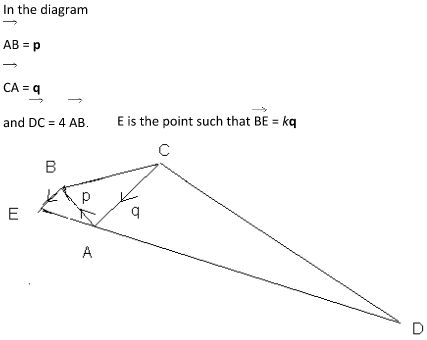Search IntMath
Close

450+ Math Lessons written by Math Professors and Teachers

5 Million+ Students Helped Each Year

1200+ Articles Written by Math Educators and Enthusiasts

Simplifying and Teaching Math for Over 23 Years

# Vectors [Solved!]

### My questionIn the diagram

AB = p

CA = q

and DC = 4 AB

E is the point such that BE = kq

d) Given that D, A and E lie on a straight line find the value of k.????

### Relevant page

3. Vectors in 2 Dimensions

### What I've done so far

a) DC+CA=DA

Hence DA = 4p + q

b) Again CB = CA+AB

Hence CB = q + p

c) Again AE = AB + BE

Therefore AE= p + kq

X

<img src="/forum/uploads/imf-triangle.jpg" width="427" height="340" alt="vectors" />

In the diagram

AB = p

CA = q

and DC = 4 AB

E is the point such that BE = kq

d) Given that D, A and E lie on a straight line find the value of k.????
Relevant page

<a href="/vectors/3-vectors-2-dimensions.php">3. Vectors in 2 Dimensions</a>

What I've done so far

a)	DC+CA=DA

Hence DA = 4p + q

b)	Again CB = CA+AB

Hence CB = q + p

c)	Again AE = AB + BE

Therefore AE= p + kq

## Re: Vectors

@Swalay: What can you tell me about the lines AB and DC?

How about the lines CA and BE?

What can we conclude about the 2 triangles ?DCA and ?ABE?

X

@Swalay: What can you tell me about the lines AB and DC?

How about the lines CA and BE?

What can we conclude about the 2 triangles ?DCA and ?ABE?

## Re: Vectors

AB and DC are parallel

CA and BE are parallel

Triangles DCA and ABE are congruents

Thank you for your help so far

AB/BE=DA/CA

(P+kq)/kq = (4p+q)/q

k=1/4

X

AB and DC are parallel

CA and BE are parallel

Triangles DCA and ABE are congruents

Thank you for your help so far

AB/BE=DA/CA

(P+kq)/kq = (4p+q)/q

k=1/4

## Re: Vectors

Actually, the triangles aren't congruent (which means same size, same shape), but similar (same shape, different size).

X

Actually, the triangles aren't <b>congruent</b> (which means same size, same shape), but <b>similar</b> (same shape, different size).

Your final answer is correct!

You need to be logged in to reply.

## Related Vectors questions

• differentiating xihat+yjhat+zkhat. [Solved!]
How is it done in a simpler example. (x^2+y^2+z^2) times [xihat+yjhat+zkhat divided by sqrt (x^2+y^2+z^2)]
• Vectors [Solved!]
In the figure (also at http://prnt.sc/cqth0m), vector OB is three times longer than OA, BC...
• Vector from angle [Solved!]
I have the angles representing the suns position on the sky. 170 degrees south 35 degrees elevation Now,...
• Vectors question [Solved!]
Let U,V & W be 3 vectors,which of the following make sense and which...